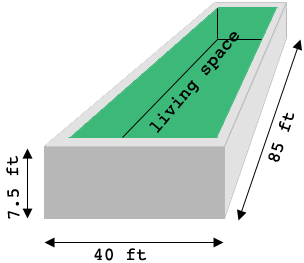SEARCH HOMEMath Central Quandaries & QueriesQuestion from Lorinda: How do you calculate ( in cubic yards) how much concrete is needed for the foundation of a rectangular building 40 ft by 85 ft if the foundation walls are 8 inches thick and 7 ft 6 inches high?Hi Lorinda,

I drew a diagram of your foundation, not to scale, with the living space having green walls and floor.The outside dimensions are 85 ft by 40 ft by 7.5 ft so that's a volume of

$85 \times 40 \times 7.5 = 25,500 \mbox{ cubic feet.}$

To determine the volume of the walls I have to subtract the volume of the living space. The walls are 8 inches thick which is 0.667 ft so the volume of the living space is

$(85- 2 \times 0.667) \times (40 - 2 \times 0.667 ) \times 7.5 = 24263.140 \mbox{ cubic feet.}$

Hence the volume of the walls is 25,500 - 24263.140 = 1,236.86 cubic feet.

You can use the internet to determine the volume in cubic yards, Just type What is 1,236.86 cubic feet in cubic yards into the Google search window or, since I'm a Mac user I would say Hey Siri, what's 1,236.86 cubic feet in cubic yards.

I didn't include the floor in my calculations. If you want to include the volume of the floor you need to add the length of the living space times its width times the thickness of the floor in feet.

I hope this helps,
PennyMath Central is supported by the University of Regina and The Pacific Institute for the Mathematical Sciences.